Your browser does not support the IFRAME tag.Home MonkeyNotes Printable Notes Digital Library Study Guides Study Smart Parents Tips College Planning Test Prep Fun Zone Help / FAQ How to Cite New Title Request

 (b) The general parabolas either in forms y2 = ax2 + bx + c or x = a1y2 + b1y + c1 (when axis of parabola is parallel to the co-ordinate axis). Now consider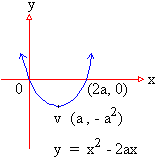i)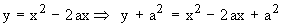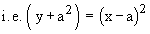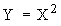It is symmetric about the New y-axis and its vertex is ( a, - a2 ).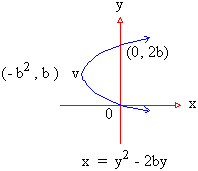ii)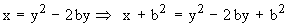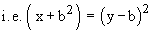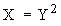which is symmetric about the New x-axis and vertex ( -b2, b ). (4) Standard Ellipse : The general equation of the ellipse in standard form is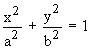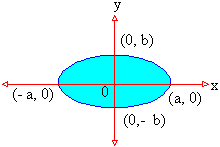(5) Standard Hyperbola : The general equation of the standard hyperbola is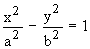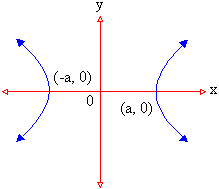Index 8.1 Introduction8.2 Area 8.3 Volumes 8.4 Mean Value 8.5 Arc Length(Rectification) Chapter 1